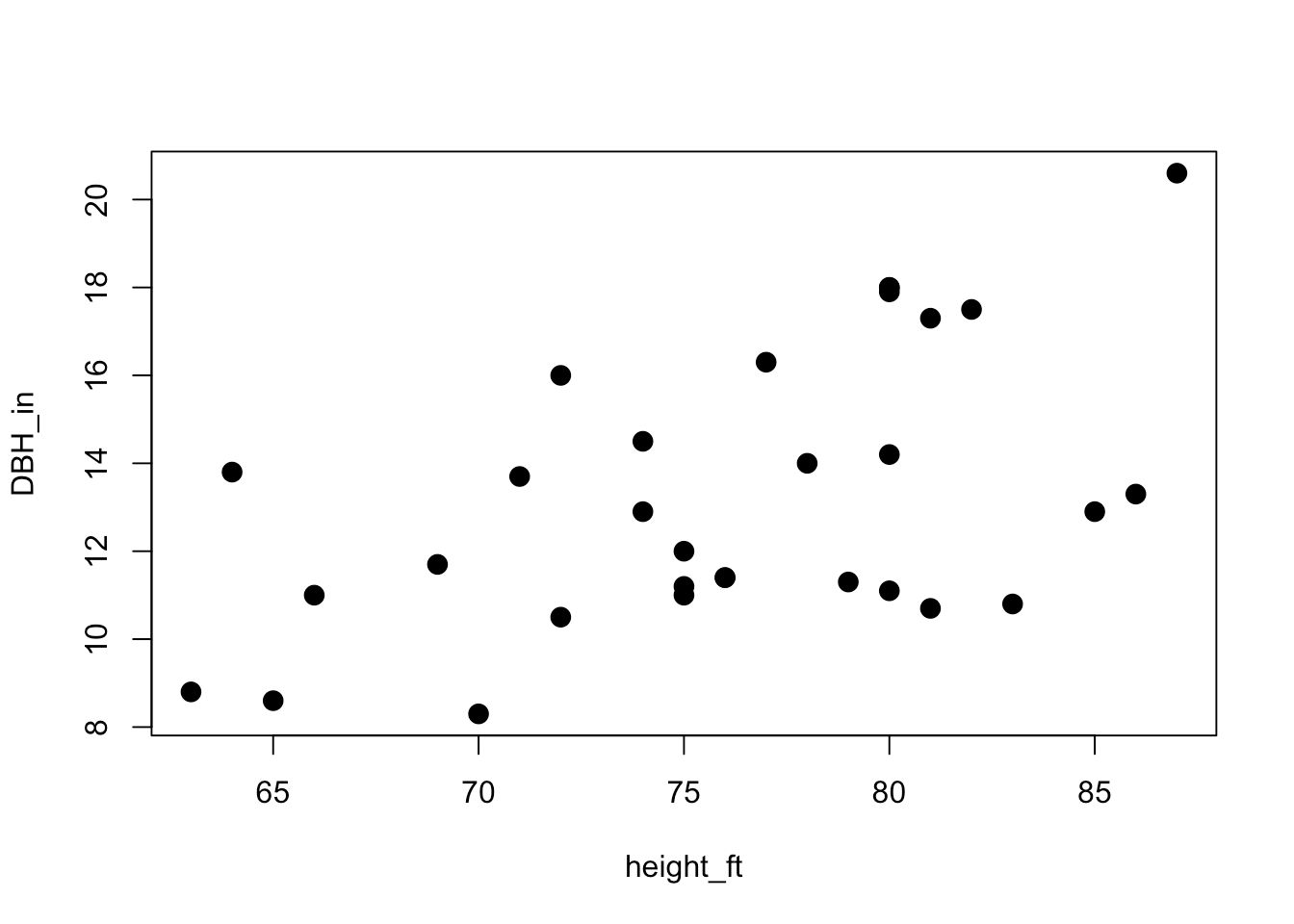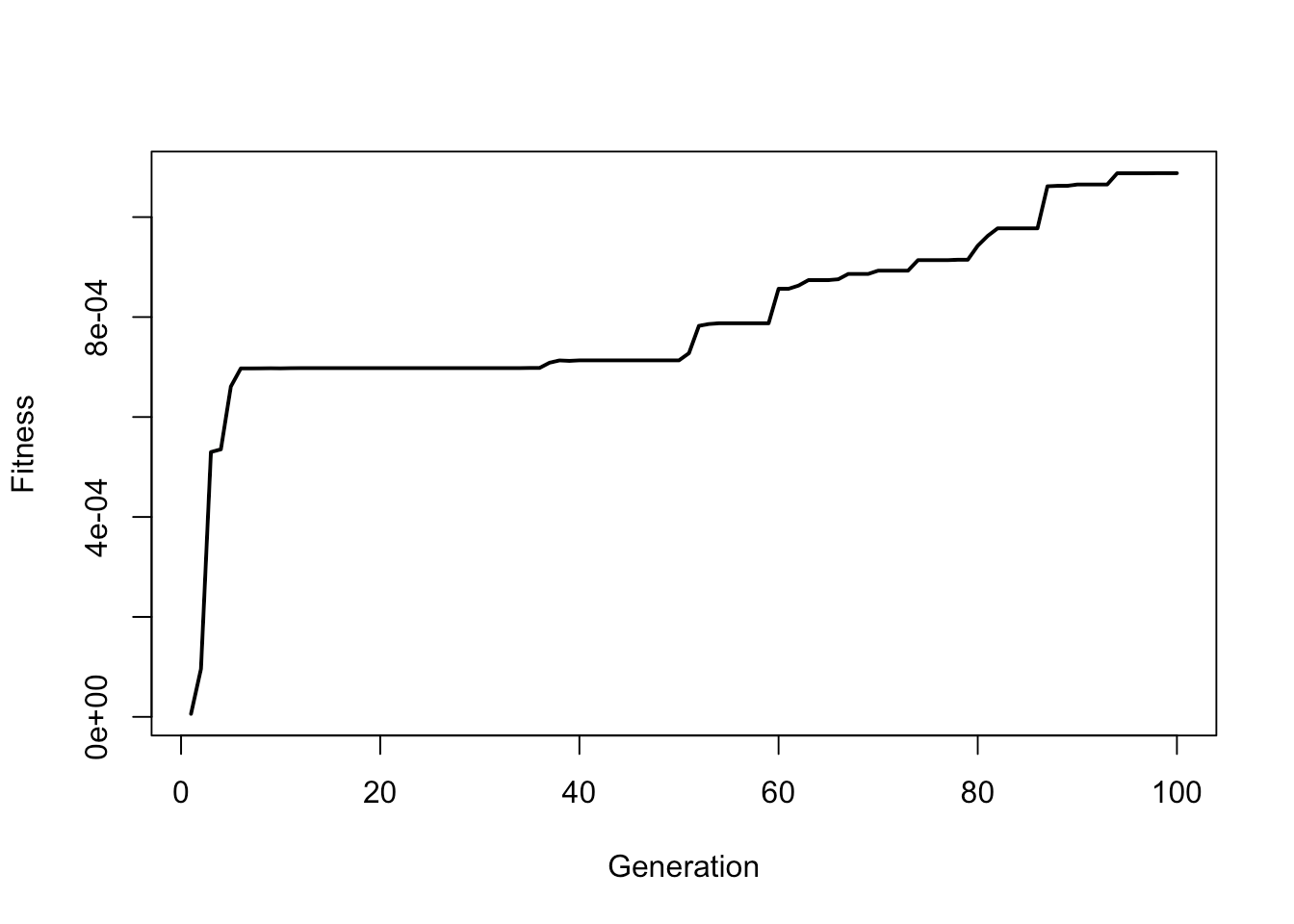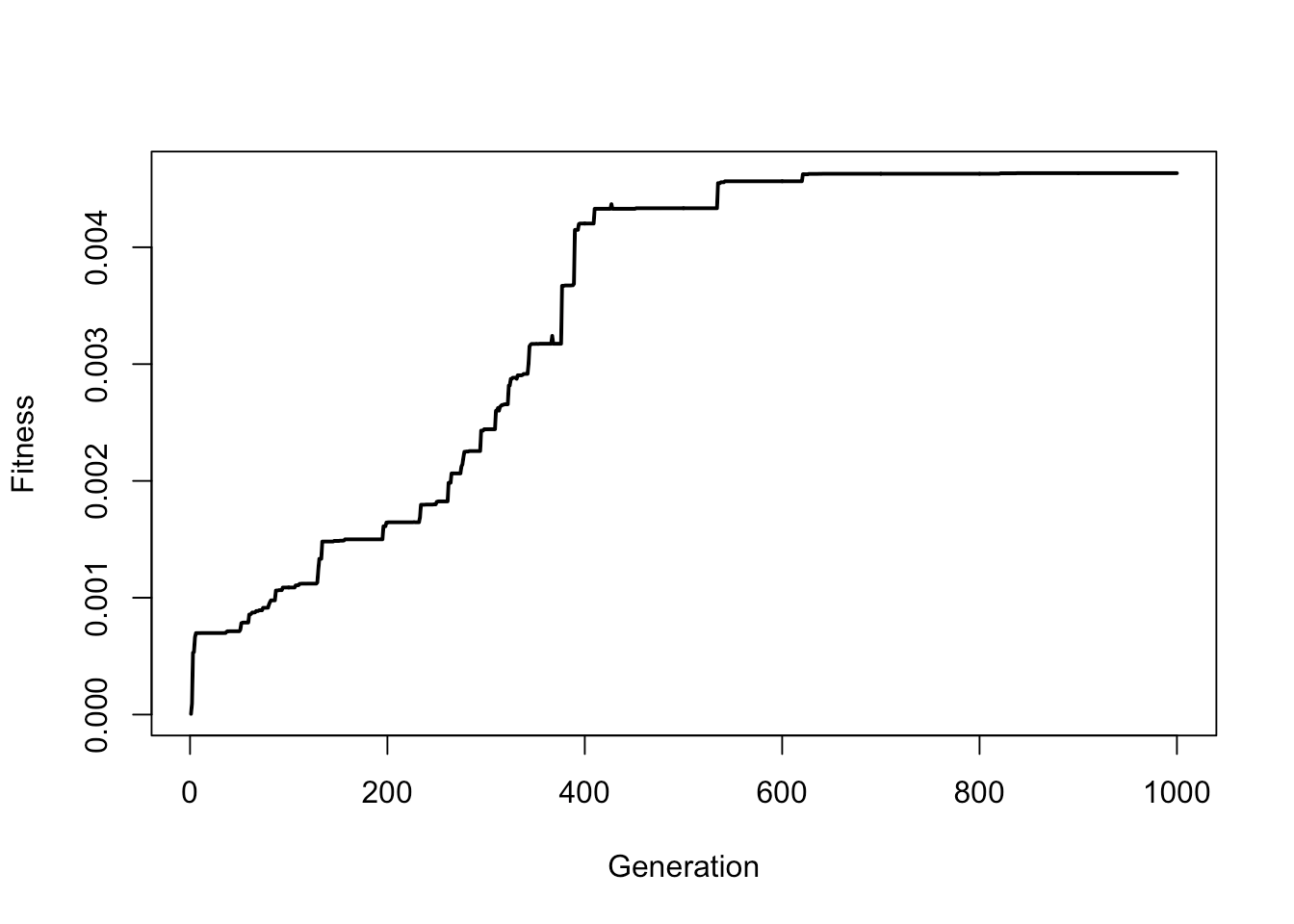Want to share your content on R-bloggers? click here if you have a blog, or here if you don't.

Evolution by natural selection is a powerful process that leads to (and continues to shape) the wonderful diversity of organisms on Earth today. It is fairly simple in its mechanics—essentially an optimization routine that allows species to evolve to an ever-changing environment:

In this intro series of posts on the basics, I want to show you how you can use the same evolutionary optimization algorithm to ‘evolve’ (optimize) solutions to other problems. Using evolutionary algorithms to solve problems is very powerful—just think of how many different solutions to flight have been reached through biological evolution.

There are all sorts of really cool applications for evolutionary algorithms, including some fun ways of simulating biological evolution itself. Jeffrey Ventrella is an algorithmic artist that uses various types of coded algorithms to create some really outstanding works of art. Of particular interest for me as an ecologist and biologist was his creation of Gene Pool—an artificial life ecosystem of swimming creatures that slowly evolve through natural selection. Check out the Swimbots website to learn more about that and see his simulation in action. Full disclosure, I’ve been recently collaborating with him on some really interesting projects aimed at studying the evolution and co-existence of swimbot creatures in Gene Pool (click here to see a video about our latest work).

For now, let’s back down a bit and focus on something somewhat simpler than virtual swimmers or flight… We’ll start with how to use evolutionary algorithms for fitting a basic linear model. We’ll use the same dataset that I went over in my tutorial for basic linear regression so that you can compare the two methods.

The basic evolutionary algorithm we use is very similar to the biological algorithm of evolution by natural selection, but I’ll expand it a bit in more detail and explain each step. I’ll note that there are some packages and functions built for running evolutionary algorithms in R, but I want to show you how it’s done from scratch so that you can understand the mechanics more directly.

To start, let’s load the data we are working with first using the `data()` function.

```# Load the data:
data(trees)
# rename columns
names(trees) <- c("DBH_in","height_ft", "volume_ft3")
# Show the top few entries:

## DBH_in height_ft volume_ft3
## 1 8.3 70 10.3
## 2 8.6 65 10.3
## 3 8.8 63 10.2
## 4 10.5 72 16.4
## 5 10.7 81 18.8
## 6 10.8 83 19.7
```

These data include measurements of the diameter at breast height in inches (DBH_in), height in feet (height_ft) and volume in feet cubed (volume_ft3) of 31 black cherry trees. I just went ahead and renamed those columns for clarity.

For this tutorial (as with my tutorial on simple linear regression), our goal is to model the association between tree height and diameter.

### `DBH_in ~ height_ft`

A quick plot shows that there is probably a relationship there:

```plot(DBH_in ~ height_ft, data = trees, pch=16, cex=1.5)
```So our goal is to determine the coefficients of the relationship between height and DBH. In other words, we want to find the best-fit line for this regression.

The actual function for the line we want is Y = a + b*X, where Y is `DBH_in`, X is `height_ft`, ‘a’ is the intercept, and ‘b’ is the slope of the line. Our goal is to ‘evolve’ a solution for the ‘a’ and ‘b’ coefficients that generate the best-fit line.

## 1) Starting Population

First we’ll create a function that generates a starting population of 100 potential organisms (models):

```set.seed(123) # to get the same results as me
# 100 random 'a' values based on a uniform distribution from -100 to 100
a_coef <- runif(min=-100, max=100, n=100)
# 100 random 'b' values based on a uniform distribution from -100 to 100
b_coef <- runif(min=-100, max=100, n=100)
# pair these together into a dataframe of two columns and call this a population
# and also add in a column for fitness so that we can keep track of the fitness
# of each organism/model:
population <- data.frame(a_coef, b_coef, fitness=NA)

## a_coef b_coef fitness
## 1 -42.48450 19.997792 NA
## 2 57.66103 -33.435292 NA
## 3 -18.20462 -2.277393 NA
## 4 76.60348 90.894765 NA
## 5 88.09346 -3.419521 NA
## 6 -90.88870 78.070044 NA
```

Now put this code into its own function:

```gen_starting_pop <- function(){
# 100 random 'a' values based on a uniform distribution from -100 to 100
a_coef <- runif(min=-100, max=100, n=100)
# 100 random 'b' values based on a uniform distribution from -100 to 100
b_coef <- runif(min=-100, max=100, n=100)
# pair these together into a dataframe of two columns and call this a population
# and also add in a column for fitness so that we can keep track of the fitness
# of each organism/model:
population <- data.frame(a_coef, b_coef, fitness=NA)
return(population)
}
```

## 2) Function to evaluate fitness

Next we need to create a function that will evaluate the ‘fitness’ of each ‘organism’ (I’ll just refer to the organisms as the ‘models’ from here on). The fitness we need in this case is based on how good of a fit each model provides. To think in terms of biological evolution, imagine that the line that the coefficients create is the phenotype while the coefficients themselves are the DNA. Natural selection always acts on the phenotype, which we can create from the DNA by applying the full model I described before: `DBH_in = a + b*height_ft`. We can evaluate the fitness by testing how well the right side of the equation predicts the left side (DBH_in).

So first we’ll loop through each model in the population to calculate how well it can predict DBH values. Then we can subtract the real DBH values from the predicted values to get the net difference between the two. Bigger differences means poor model and lower fitness. To create just one ‘fitness’ value per model, we can then square and sum those difference values together. This generates an overall index of how different the predicted DBH is from the real DBH. The reason we square the values is to count negative and positive differences in the same way (only absolute difference is what matters). Finally to make the fitness value range from 0 to 1, with 1 being the perfect fitness (which is actually impossible in our case), simply inverse the result (1/result):

```# loop through each organism:
for(i in 1:100){
# for each organism, calculate predicted DBH values
DBH_predicted = population[i,"a_coef"] + population[i,"b_coef"]*trees\$height_ft
# Now, subtract the real DBH values from the predicted values to get the difference:
difference <- DBH_predicted - trees\$DBH_in
# calculate the sum of squared differences:
sum_sq_diff <- sum(difference^2)
# make the fitness value range from 0 to 1:
fitness <- 1/sum_sq_diff
# finally, save the fitness values to the population dataframe:
population\$fitness[i] <- fitness
}
```

Then we need to choose survivors. Let’s say only the top 10 models with the highest fitness will survive:

```# find the index of the top 10 (highest) fitness values:
top_10_fit <- order(population\$fitness, decreasing = T)[1:10]
# then use those to index the population:
survivors <- population[top_10_fit,]
survivors

## a_coef b_coef fitness
## 61 33.02304 0.4599127 1.073925e-05
## 52 -11.55999 -0.4945466 8.224736e-06
## 5 88.09346 -3.4195206 9.275710e-07
## 3 -18.20462 -2.2773933 7.663413e-07
## 35 -95.07726 4.2271452 7.017355e-07
## 86 -13.02145 -3.7420399 3.320522e-07
## 78 22.55420 5.9671372 1.497032e-07
## 66 -10.29673 6.7375891 1.342400e-07
## 96 -62.46178 -6.6934595 9.392552e-08
## 17 -50.78245 9.8569312 6.820099e-08
```

But let’s also put this code that calculates fitness and picks survivors into its own function to make it easier to write out in future steps:

```evaluate_fitness <- function(population){
# loop through each organism:
for(i in 1:100){
# for each organism, calculate predicted DBH values
DBH_predicted = population[i,"a_coef"] + population[i,"b_coef"]*trees\$height_ft
# Now, subtract the real DBH values from the predicted values to get the difference:
difference <- DBH_predicted - trees\$DBH_in
# calculate the sum of squared differences:
sum_sq_diff <- sum(difference^2)
# make the fitness value range from 0 to 1:
fitness <- 1/sum_sq_diff
# finally, save the fitness values to the population dataframe:
population\$fitness[i] <- fitness
}
# find the index value the top 10 (highest) fitness values:
top_10_fit <- order(population\$fitness, decreasing = T)[1:10]
# then use those to index the population:
survivors <- population[top_10_fit,]
# return survivors
return(survivors)
}
```

Now, all we have to do is call:
`population <- evaluate_fitness(population)`
whenever we want to evaluate the survivors of the population.

## 3) Mate survivors and mutate DNA (and generate the new population)

Next we need to create a new population of 100 models using those survivors, making sure to add some random mutations to ensure the potential for evolution exists.

First, generate the new population of models by cloning these survivors at random:

Note, I’m just cloning individuals rather than sexual reproduction here to keep things simple for this example. However, DNA crossover can provide an important advantage for evolutionary algorithms as it does in biology.
``` # First, choose the parents at randome from the 10 possible survivors:
parents <- sample(1:10, size=100, replace=T)
# and use those parents (index values) to clone offspring:
offspring <- survivors[parents,]
# choose a mutation rate:
mutation_rate <- 0.6
# total number of mutations is our population (100) * the rate, and rounded to
# make sure the result is an integer value:
total_mutations <- round(100*mutation_rate)
# choose which models recieve mutations for a or b coefficients:
a_to_mutate <- sample(x=c(1:100), size=total_mutations)
b_to_mutate <- sample(x=c(1:100), size=total_mutations)
# then generate a set of random mutations for the a and b coefficients:
a_mutations <- rnorm(n = total_mutations, mean=0, sd=3)
b_mutations <- rnorm(n = total_mutations, mean=0, sd=3)
# and apply those mutations:
offspring\$a_coef[a_to_mutate] <- offspring\$a_coef[a_to_mutate] + a_mutations
offspring\$b_coef[b_to_mutate] <- offspring\$b_coef[b_to_mutate] + b_mutations
# finally, reset the row names from 1 to 100:
row.names(offspring) <- 1:100
```
Note, I’m setting the mutation rate to 0.6 and the magnitude for the mutations to 3 (`sd=3`), but you can play around with mutation rate and magnitude to see what happens.

But let’s also make this step into a function so that we can easily call it later when we are looping through each generation:

```mate_and_mutate <- function(survivors){
# create a series of 100 random indexes chosen from the survivors:
# First, choose the parents at randome from the 10 possible survivors:
parents <- sample(1:10, size=100, replace=T)
# and use those parents (index values) to clone offspring:
offspring <- survivors[parents,]
# choose a mutation rate:
mutation_rate <- 0.6
# total number of mutations is our population (100) * the rate, and rounded to
# make sure the result is an integer value:
total_mutations <- round(100*mutation_rate)
# choose which models recieve mutations for a or b coefficients:
a_to_mutate <- sample(x=c(1:100), size=total_mutations)
b_to_mutate <- sample(x=c(1:100), size=total_mutations)
# then generate a set of random mutations for the a and b coefficients:
a_mutations <- rnorm(n = total_mutations, mean=0, sd=3)
b_mutations <- rnorm(n = total_mutations, mean=0, sd=3)
# and apply those mutations:
offspring\$a_coef[a_to_mutate] <- offspring\$a_coef[a_to_mutate] + a_mutations
offspring\$b_coef[b_to_mutate] <- offspring\$b_coef[b_to_mutate] + b_mutations
# finally, reset the row names from 1 to 100:
row.names(offspring) <- 1:100
# return the new generation of offspring:
return(offspring)
}
```

So that was one generation, great! Now we need to repeat the cycle for many more generations. We’ll put everything together with the help of the `for` loop.

```# First set the starting population:
population <- gen_starting_pop()
# set how many generations you want to run this for.
generations <- 5
# begin the for loop:
for(i in 1:generations){
# 1) Evaluate fitness:
survivors <- evaluate_fitness(population)
# 2) Mate and mutate survivors to generate next generation:
next_generation <- mate_and_mutate(survivors)
# 3) Redefine the population using the new generation:
population <- next_generation
}
survivors <- evaluate_fitness(population)

## a_coef b_coef fitness
## 46 -13.872104 0.3557666 0.004382764
## 95 -14.649663 0.3592565 0.004170481
## 8 -3.179754 0.2292144 0.004046204
## 67 -5.458559 0.2292144 0.003732053
## 11 -15.186570 0.3557666 0.003467042
## 59 -12.313690 0.3557666 0.003383982
```

Notice how even in just a few generations the fitness has gone up quite a bit. We can actually plot and visualize how the fitness changes over time by saving a fitness value from each generation:

```set.seed(1239)
# First set the starting population:
population <- gen_starting_pop()
# set how many generations you want to run this for.
# Use 100 now:
generations <- 100
# define empty variable to collect fitness values:
fitness <- NULL
# begin the for loop:
for(i in 1:generations){
# 1) Evaluate fitness:
survivors <- evaluate_fitness(population)
# 2) Mate and mutate survivors to generate next generation:
next_generation <- mate_and_mutate(survivors)
# 3) Redefine the population using the new generation:
population <- next_generation
# save fitness value from each generation to plot it over time
fitness[i] <- max(population\$fitness)
}
```

And plot the results during the first 100 generations:

```plot(fitness ~ c(1:generations), type="l", lwd=2, xlab="Generation", ylab="Fitness")
```So you can see how fitness slowly increases over time. Try this again but run the simulation for 1000 generations (note, that it may take a minute to run the for loop):We can also visualize the evolution of the best-fit line by plotting how the line changes over time. The code below runs the first 100 generations and every 5 generations it pauses to plot the scatterplot with the predicted line:

```set.seed(1239)
# First set the starting population:
population <- gen_starting_pop()
# set how many generations you want to run this for.
generations <- 1000
# define empty variable to collect fitness values:
fitness <- NULL
# begin the for loop:
for(i in 1:generations){
# 1) Evaluate fitness:
survivors <- evaluate_fitness(population)
# 2) Mate and mutate survivors to generate next generation:
next_generation <- mate_and_mutate(survivors)
# 3) Redefine the population using the new generation:
population <- next_generation
fitness[i] <- max(population\$fitness)
#Every 5 generations, pause the simulation and plot the
# points with the current best-fit line:
if(i %% 50 == 0){
survivors <- evaluate_fitness(population)
plot(DBH_in ~ height_ft, data = trees, pch=16, cex=1.5)
title(main=paste0("Generation: ",i), cex.main=2)
abline(a=survivors\$a_coef, b=survivors\$b_coef, lwd=3, col="red")
# pause for 1 second:
Sys.sleep(.5)
}
}
```

Now let’s extract the highest fitness model after having run the simulation for 1000 generations to see how the coefficients compare to the basic linear model `lm()` output in R:

```# Extract the best model:
top_models <- evaluate_fitness(population) # Be sure to run this first
best_model <- top_models[which.max(top_models\$fitness),]
# compare this to a basic linear model result with 'lm()':
evo_model <- best_model
lm_model <- lm(DBH_in ~ height_ft, data=trees)
# Plot the scatterplot and add the best-fit lines:
plot(DBH_in ~ height_ft, data = trees, pch=16, cex=1.5)
# red line for our model solution
abline(a=evo_model\$a_coef, b=evo_model\$b_coef, col="red", lwd=2)
# blue line for the lm() model solution:
abline(lm_model, col="blue", lwd=2)
```Not bad for only 1000 generations! Can barely even see the difference between the two lines.

### Conclusion:

our model: a = -5.9599 b = 0.2528
lm() model: a = -6.1884 b = 0.2557

So, those are the basics of how evolutionary algorithms work. I hope this part of the tutorial was helpful. In the next part I’m going to get a bit more advanced and show you how I used an evolutionary algorithm to create images made of letters such as the one below.

If you liked this post and want to learn more, then check out my online course on the complete basics of R for ecology:

Also be sure to check out R-bloggers for other great tutorials on learning R